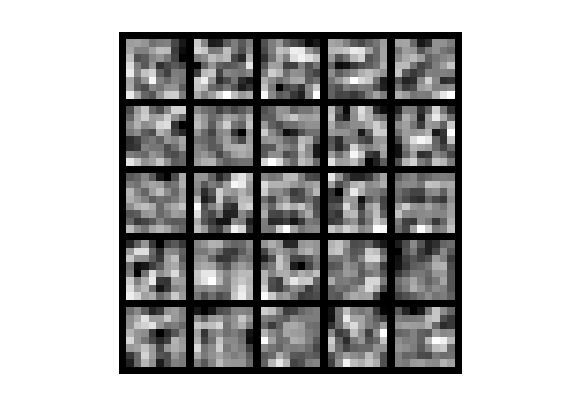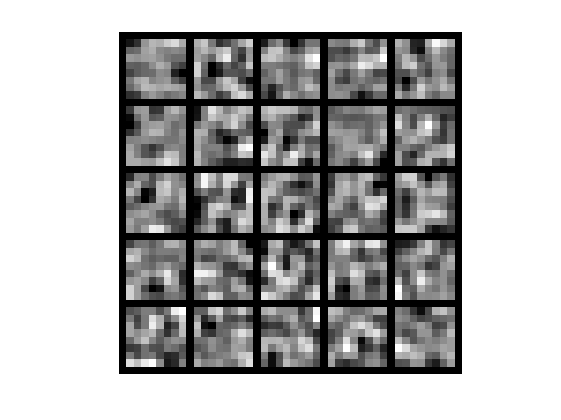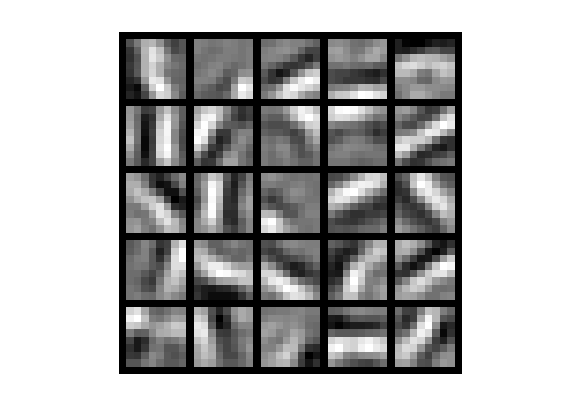# 稀疏自编码器及其实现——如何搞基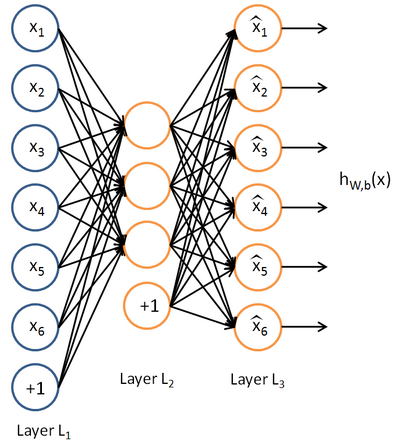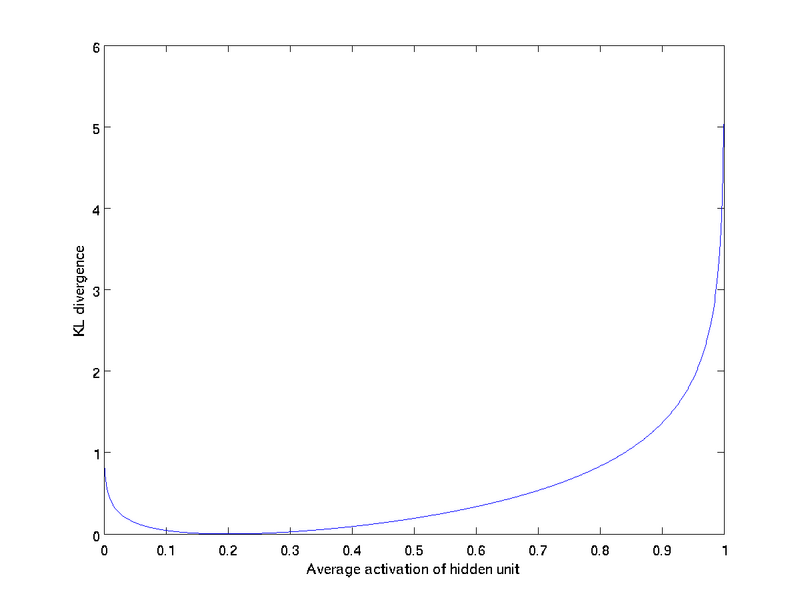<span style="font-size:14px;">loadIMAGES;
imagesc(IMAGES(:,:,6))
colormapgray;</span>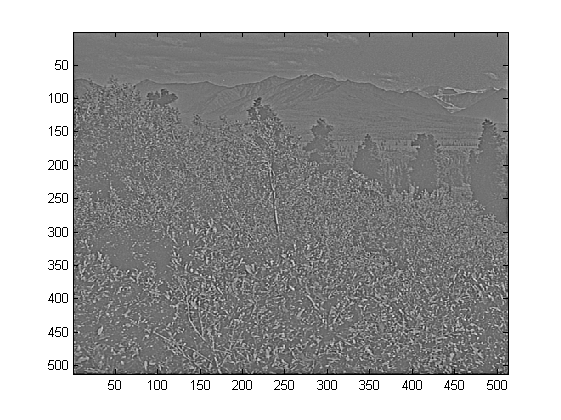<span style="font-size:14px;">loadIMAGES;    % load images from disk
patchsize= 8;  % we'll use 8x8 patches
%numpatches = 10;
numpatches= 10000;
%Initialize patches with zeros.  Your codewill fill in this matrix--one
%column per patch, 10000 columns.
patches= zeros(patchsize*patchsize, numpatches);
tic
image_size=size(IMAGES);
i=randi(image_size(1)-patchsize+1,1,numpatches);
j=randi(image_size(2)-patchsize+1,1,numpatches);
k=randi(image_size(3),1,numpatches);
fornum=1:numpatches
patches(:,num)=reshape(IMAGES(i(num):i(num)+patchsize-1,j(num):j(num)+patchsize-1,k(num)),1,[]);
end
toc</span>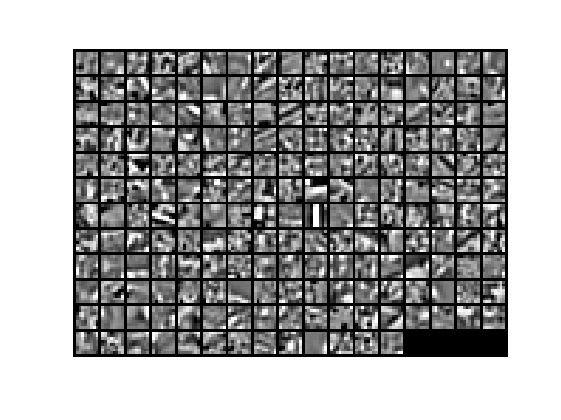sparseAutoencoderCost.m

<span style="font-size:14px;">numpatches=size(patches,2);
a2=sigmoid(W1*patches+repmat(b1,1,numpatches));
a3=sigmoid(W2*a2+repmat(b2,1,numpatches));
Rho=sum(a2,2)/numpatches;
Penalty=-sparsityParam./Rho+(1-sparsityParam)./(1-Rho);
Delta3=(a3-patches).*a3.*(1-a3);
Delta2=(W2'*Delta3+beta*repmat(Penalty,1,numpatches)).*a2.*(1-a2);
cost1=sumsqr(a3-patches)/numpatches/2;
cost2=(sumsqr(W1)+sumsqr(W2))*lambda/2;
cost3=beta*sum(sparsityParam*log(sparsityParam./Rho)+(1-sparsityParam)*log((1-sparsityParam)./(1-Rho)));
cost=cost1+cost2+cost3;
b1grad=sum(Delta2,2)/numpatches;</span>

<span style="font-size:14px;">EPSILON=0.0001;
thetaspslion=zeros(size(theta));
fori=1:size(theta)
thetaspslion(i)=EPSILON;
thetaspslion(i)=0;
end</span>